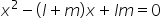Maths-
General
Easy

###Hint:

In this question, we have to find the quadratic equation where the roots l and m are given. First we will find the value of l and m. Then to find the quadratic equation when l and m are roots we will use the form.

## Book A Free Demo+91